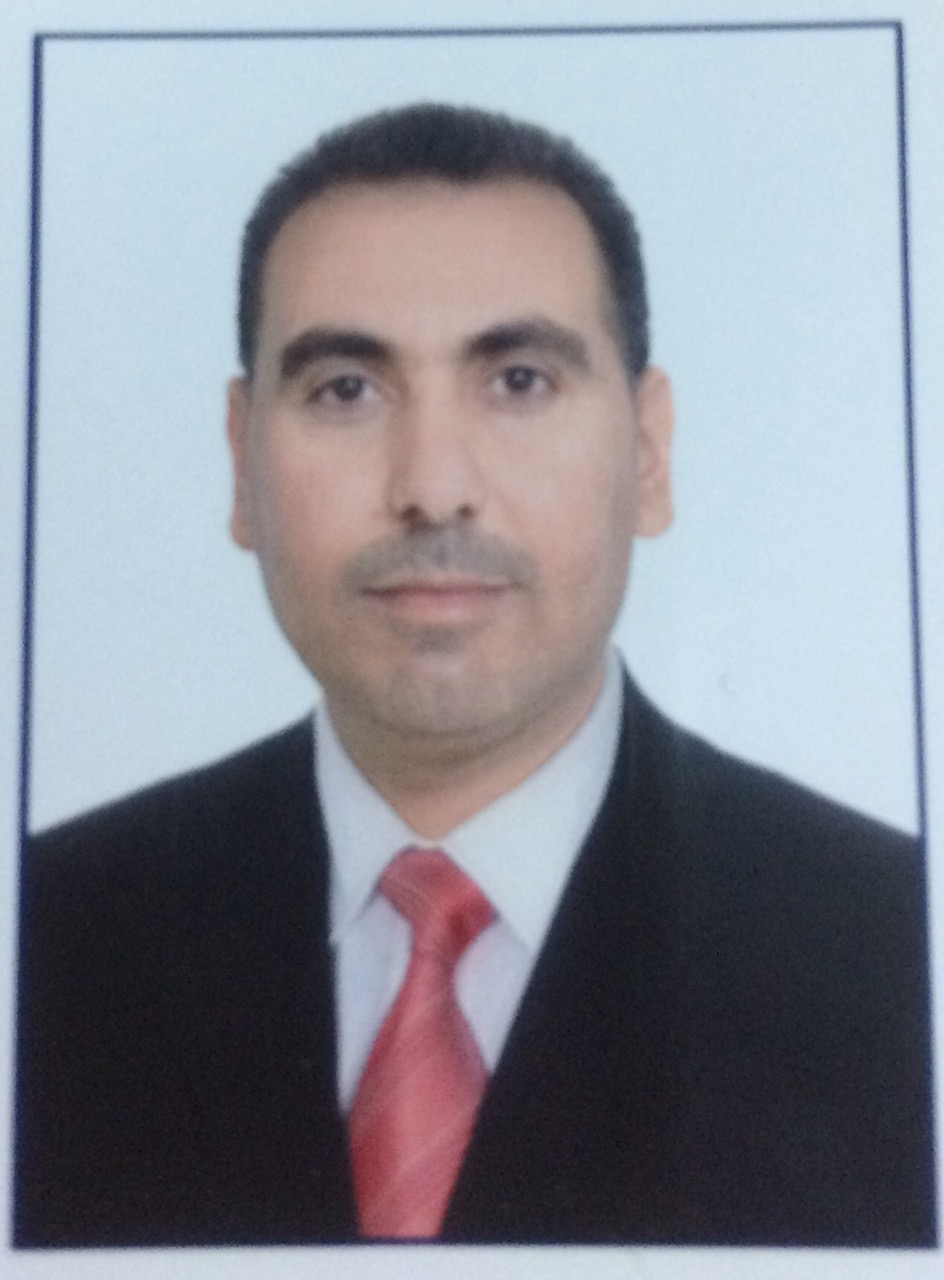BackAss. Lecturer Hussein Burhan Mohammed got on bachelor's degree in Automated Manufacturing Engineering from University of Baghdad / Iraq, 2003. He got a master's degree in mechanical engineering in major (Manufacturing& Industrial) from University of Baghdad, 2012. Now I'm working as (Ass. Lecturer) at Materials Dep./ College of Engineering/  University of Diyala

Research Interest: – (cryogenic treatment, heat treatment of steel, powder metallurgy, composite materials)

Subject Name:- mathematics I/second stage / materials Dep.,  mathematics I I/ first stage/ mechanical Dep., engineering  drawing / first stage/ materials Dep

Weekly schedule:-

 Time stage subject Today :1130- 1:30 Second Materials Dep. Advanced Mathematics Sunday 10:30- 12:30 First Mechanical Dep. Mathematics I Monday 8:30- 12:30 First Materials Dep. Engineering drawing Tuesday 11:30- 9:30 first Mechanical Dep. Mathematics I Wednesday 10:30- 8:30 Second Materials Dep. Advanced mathematics Tuesday

 First stage Dep. of mech. (Mathematics) Lectures Title of Lectures Lectures one Inequality, absolute value & functions Lectures two Composition of functions, shifting of graph Lectures three Trigonometric functions, limits & continuity Lectures four Differentiation & higher derivatives Lectures five Derivative of trigonometric functions and chain rule Lectures six Applications of differentiation& relative extreme points Lectures seven Graph of functions Lectures eight Rolls rule and M. V. T. Lectures nine Integration Lectures ten Finite integration I Lectures eleven Integration by substitution Lectures twelve Area under the curve Lectures thirteenth Volumes , disk method Lectures fourteenth Washer method Lectures fifteenth Cylindrical method Lectures sixteenth Surface area Lectures seventeenth Hyperbolic functions Lectures eighteenth Logarithmic functions and exponent functions References Calculus Second  stage Dep. of material . (advanced mathematics) Lectures Title of Lectures Lectures one Vectors Lectures two Dot product & cross product Lectures three Vector projection Lectures four Equation of line  and plane in space Lectures five Distance between the line and point& distance between point and plane Lectures six Series Lectures seven Test convergence and divergence of series Lectures eight Test convergence and divergence of series Lectures nine Test convergence and divergence of series Lectures ten Power series Lectures eleven Using of series to evaluate of complex integrals and limits Lectures twelve Sequences Lectures thirteen Differential equations Lectures fourteenth Methods of solution of D. E. Lectures fifteenth Methods of solution of D. E. Lectures sixteenth Methods of solution of D. E. Lectures seventeenth Methods of solution of D. E. Lectures eighteenth Polar  coordinates Lectures nineteenth Area in polar  coordinates Lectures twenty Length of curve in polar coordinates References Calculus

 Lectures first course Title of Lecture First class Lectures one Introduction in engineering Drawing Lectures two Drawing equipment Lectures three Sheet plans Lectures four Types of lines Lectures five Arcs Lectures six Pentagon and hexagon Lectures seven Ellipse References Engineering Drawing book for abd Al Rasool Lectures Second course Title of Lecture First class Lectures one Projection Lectures two Third view Lectures three Dimensions Lectures four Section Lectures five Isometric

Publications:

Abbas Abdul Hussein abid, Hussein Burhan Muhammed, " Effect of Cryogenic Trteatment on the Tensile Properties of Carbon Dual Phase Steel" Journal of Engineering, No. 5, Vol. 19, may 2013